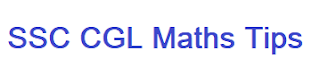# How to prepare maths for SSC CGL? SSC CGL Maths Preparation Tips

Mathematics is a scoring subject in SSC CGL and every students wants to score good marks in maths  as mathematics has sure marks, I mean after exam and during the exam we are sure that these questions are correct and we will get full marks for these questions. So here today, I will talk about how to prepare maths for ssc cgl so that we can get full marks in mathematics section.How to prepare maths for ssc cgl?

## How to prepare maths for SSC CGL?

Mathematics is a time consuming subject. Many times, in the examination, we could not solve all those questions that we can solve at home easily. So, if we want to score full marks in ssc cgl maths then we have to solve these questions within the stipulated time. These questions can only be solved within the time in following two cases and both are necessary to score full marks in ssc cgl maths:

1. Concepts should be clear mind
2. Have regular practice before exam
3. Solving previous years questions

### a. Concepts should be clear for all these math questions/ math topics

If we want to score full marks in SSC CGL maths then concepts of mathematics for each topics, which is asked in ssc cgl exam, should be clear. Some books like Mathematics by R.S. Agrawal, Lucent's Higher Mathematics etc. are very helpful for preparation of mathematics. Some topics are very common and we can solve them by using our sense like number system, age related questions, profit and loss etc. but some topics like trigonometry and some topics in geometry are formula based, for which we have to remember those formula. These are base of our preparation, so we should focus on these points and understand the basic concepts of it.How to prepare maths for ssc cgl

### b. Regular practice for attempting the questions within the time.

Practice makes man perfect therefore practice is very important. By practice, we can attempt more question within the time. After every practice, we should note down which question taken more time and why, we should find out the reason why it taken more time and also find out easy way to solve these types of questions within the prescribed time. Many times we find that only practice is the easiest way to solve the questions within time.

How to clear SSC CGL in First Attempt?: Read Here

### C. Solving previous years questions

Solving previous years questions is very important as many questions are repeated from previous papers. Also this helps in understanding the pettern of questions which are asked in previous exams. So, solve at least 5 years previous years question paper before appearing in the exam.

SSC CGL Tier 3 Previous Years Papers: Get Here

### How to prepare maths for SSC CGL? SSC CGL Maths Preparation Tips:

In addition to this solve previous years question papers of mathematics for ssc cgl because the pattern of the questions in ssc cgl exam are almost same every year. The other benefit of solving previous years question papers is, that at least 30-35% of questions of previous years are asked as it is in ongoing exams.

SSC CGL Exams are being conducted on computer, so solve at least one practice set every day on Computer so that we become families with the Computer Based Exams.

The above article on How to prepare maths for ssc cgl, is based on my own experience. You can tell me your views on this in comment section.

Best of luck for your exam.

How to crack SSC CGL in first attempt?

20 Most important essay topics for SSC CGL Tier 3

How to prepare maths for SSC CGL?  SSC CGL Maths Preparation Tips

1.2.